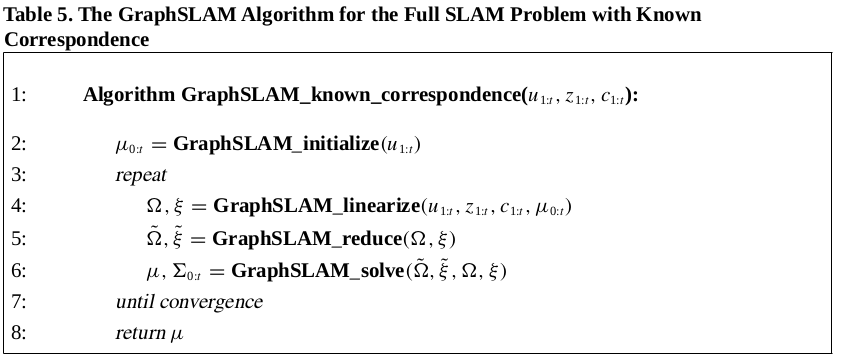0%

### 位姿和地图特征点求解

• 通过控制量 $u{t_1+1}, u{t1+2}, …, u{t2-1}, u{t_2}$ 连接
• 通过 $m_j$ 连接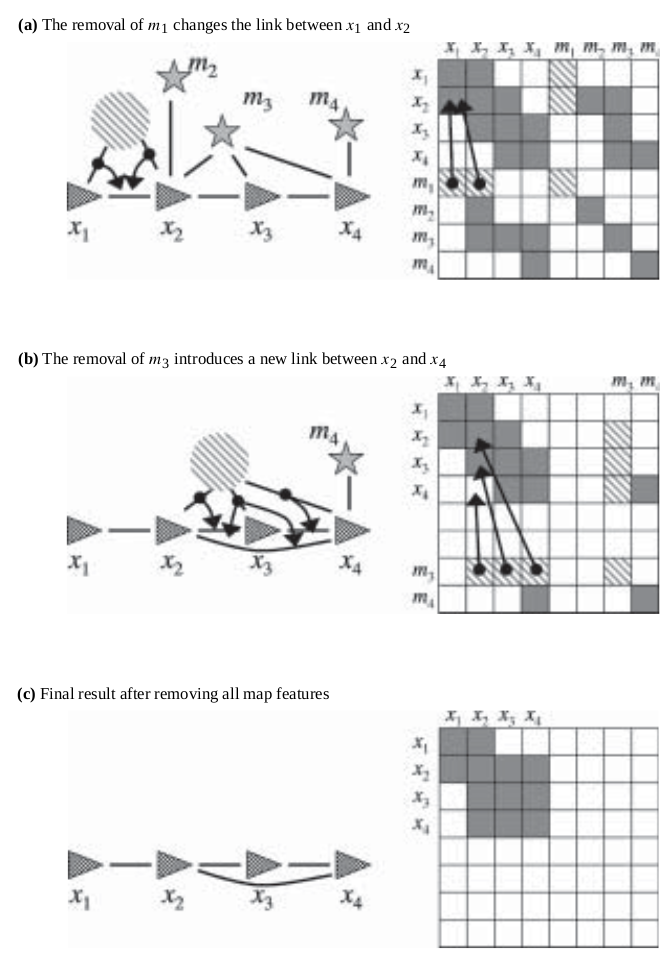• $x_t\in \tau(j) \Leftrightarrow \exist i: c_t^i = j$

$ct$ 是位姿观测到的所有特征点序号。根据这个定义，对于特征点 $m_j$ 而言，我们知道它只会跟 $\tau(j)$ 中的位姿 $x_t$ 产生连接，而不会跟其他位姿产生连接。那么我们可以通过对 $\tau(j)$ 中任意两个位姿 $x_t, x{t’}$ 添加连接，从而将 $m_j$ 和 $\tau{j}$ 中所有连接去除（在信息矩阵和信息向量相应位置的值设为 0）。值得注意的是，这个操作只是对信息矩阵进行局部的修改（时间花费不高）。在去除掉所有特征点之后，信息矩阵 $\Omega$ 和信息向量 $\xi$ 的维度会大大降低（因为缺少了所有特征点），而对于位姿节点来说，解（或者说约束）仍然是等效的。

• 构建图：向一个大的信息矩阵根据每一次观测和每一次控制输入通过线性化约束加入局部信息（小矩阵）
• 通过去除特征点并在特征点之间引入新的约束降低矩阵维度
• 求解机器人位姿
• 通过解出来的机器人位姿反求特征点位置

### GraphSLAM 算法

#### 解的初始化

• $t = 0$, 直接初始化为0，即： $\mu = (0, 0, 0)^T$
• $t > 0$ 的情况则利用运动模型函数进行链式计算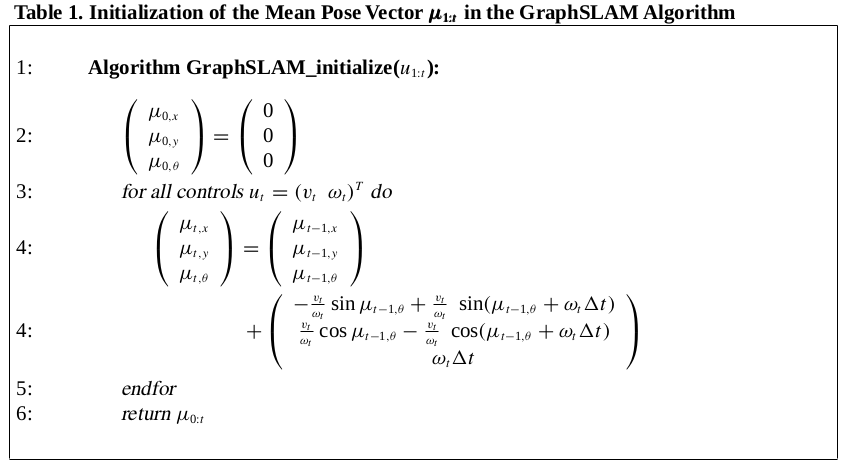#### 构建信息矩阵 $\Omega$ 和信息向量 $\xi$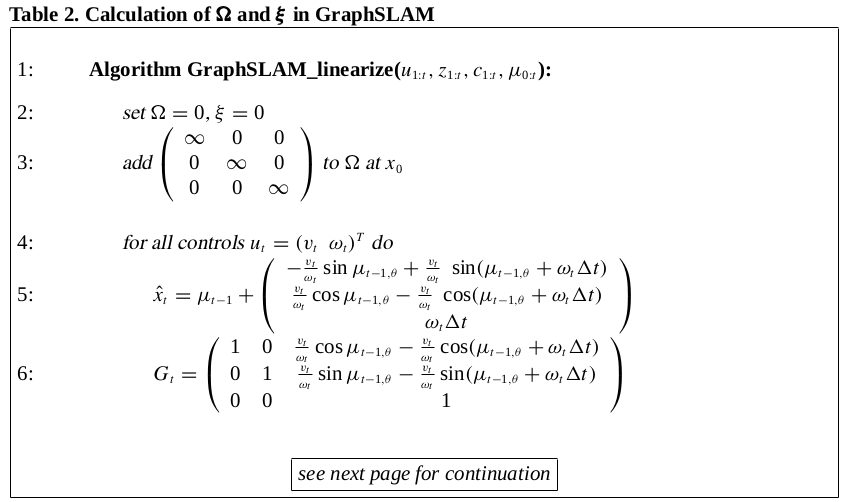• 控制输入：$u_{1:t}$
• 观测值：$z_{1:t}$
• 观测值对应的相关系数：$c_{1:t}$
• 位姿初值：$\mu_{0:t}$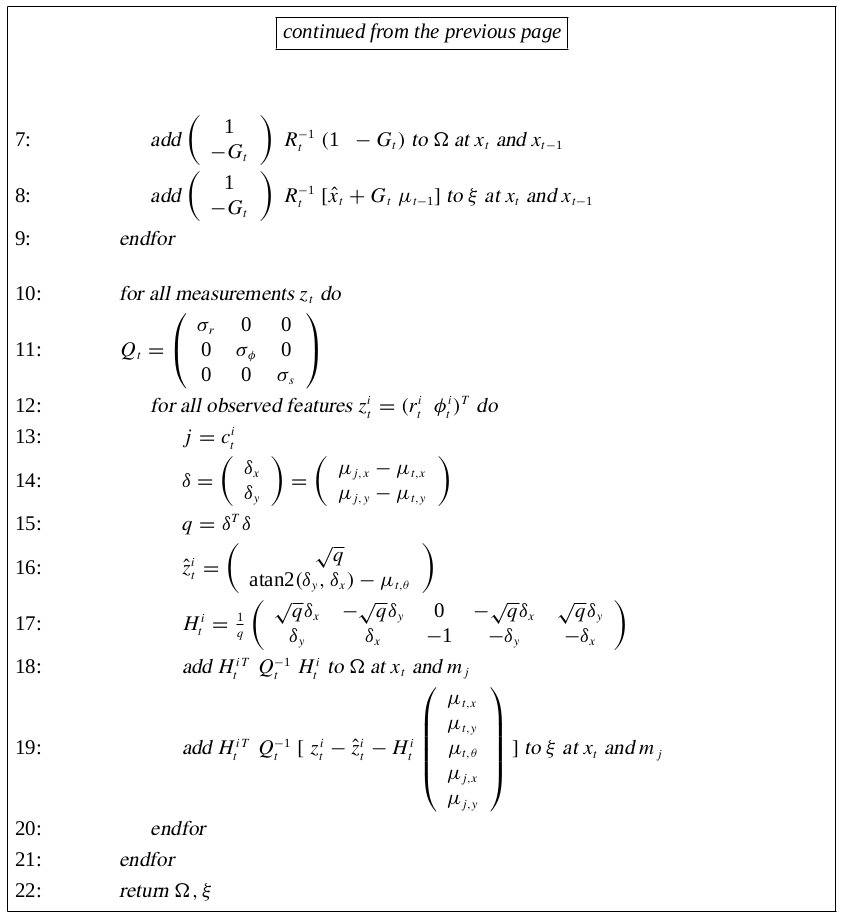#### 简化图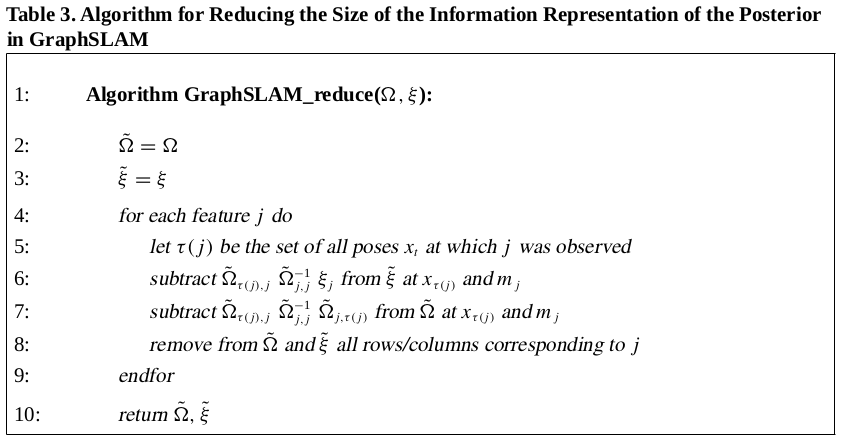• （第 5 行）找出观测到该相关点的所有位姿集合 $\tau(j)$
• 从当前的信息向量 $\xi$ 中在 $x{\tau(j)}$ 和 $m_j$ 的位置减去 $\tilde{\Omega}{\tau(j),j}\tilde{\Omega}_{j,j}^{-1}\xi_j$
• 从当前的信息矩阵 $\Omega$ 在 $x{\tau(j)}$ 和 $m_j$ 的位置减去 $\tilde{\Omega}{\tau(j),j}\tilde{\Omega}{j,j}^{-1}\tilde{\Omega}{\tau(j),j}$
• 去掉 $\tilde{\Omega}$ 和 $\tilde{\xi}$ 中所有和 $j$ 有关联的行和列

#### 求解机器人位姿和地图特征点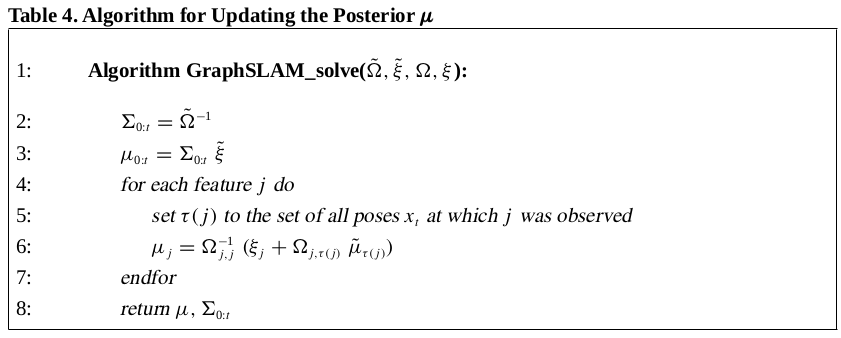GraphSLAM 算法中解的精度很大程度上取决于均值的选取。均值的选取好坏会导致泰勒展开的精度，从而影响算法精度。为了尽可能减少泰勒展开线性化的错误，可以进行迭代更新初值，即重复进行 GraphSLAM_linearizeGraphSLAM_reduceGraphSLAM_solve。每次迭代利用上一次求得的解作为初值。论文中提到只有初值错误很高（超过 20 度）的时候才有必要迭代计算，并且一般来说 3 次左右迭代次数就足够了。

#### 算法总结

1. 设初值
2. 线性化
3. 简化图
4. 求解
5. 重复 2 ～ 4 步直至收敛
6. 返回机器人位姿均值方差，和地图特征点的均值